# Lesson 6: Constructing Multiple-Decrement Life Tables

## 6.0 Overview

Lessons 1-5 introduced tools and techniques to construct multiple-decrement life tables using vital statistics data (or macro-level data) such as age and age-cause-specific death rates. Lessons 6-7 extend these ideas to construct multiple-decrement life tables using observational or follow-up (or micro-level) data. Before applying multiple-decrement life table techniques to the follow-up data (in Lesson 7), we will learn the ordinary life table concepts to analyze follow-up or observational data.

In this session you will:

1. Examine various data structures and learn several key terms, such as time to event, censoring, and lost to follow-up.
2. Learn how to apply ordinary life table concepts to analyze this data.
3. Construct ordinary life tables using two sample datasets.
4. Calculate variance and standard error of the estimated life table parameters.

Download a zip file containing data for Lesson 4 in Excel and CSV formats.

## 6.1 Data Structure

Cohort Examples and Terminology

1. A birth cohort (children born at the same time) is followed for 12 months; if a death occurs, the time of death is noted. Those who did not die will be labeled as survivors at the end of 12 months of observation.
2. A marriage cohort (all women married at the same time) is followed for a fixed period. If a marital disruption occurs, the date of this event is recorded. For those who did not have a marital disruption, the duration of their follow-up is noted.
3. A contraceptive cohort (all individuals who accepted a contraceptive at the same time) is followed for various intervals of time. For individuals who discontinued the use of contraception, the duration of use is noted. For the continuing users at the end of follow-up, the duration of follow-up is also recorded.

Terminology

Note that the focus in all three cohorts is on a particular event. In the birth cohort, the event of interest is infant death. In the marriage cohort, the event of interest is marital disruption. And in the contraceptive cohort, the event is discontinuation of the contraceptive.

In all three cohorts, the beginning point of exposure to the event is well defined. In the birth cohort, the beginning point of exposure to death is the date of birth. In the marriage cohort, it is the date of marriage, and in the contraceptive cohort, the date of contraceptive acceptance.

In all examples, the date of experiencing of the event is of interest. In the first, it is the time elapsed between dates of birth and death, if death occurs. With the second, it is the time elapsed between the date of marriage and the date of dissolution of marriage. And in the third example, it is the time elapsed between the date of acceptance of the contraception and the date of discontinuation.

The time to event (time elapsed between beginning point and the time of occurrence of event) is also calledfailure time. In the first example the time to event or the failure time is the age in months at which the infant death occurs. One can also characterize the time to infant death in days. In the second example, the failure time is the duration of the marriage at which the marriage ended. In the third example, the time to event is the duration of contraceptive use at which the individual stopped using.

In the birth cohort, some infants had survived the first year of life. In other words, these survivors time to death exceeded 12 months. Thus, the data shows two kinds of measurements. For those who died within 12 months the time to event (age at death) is known. For those who survived we know only that the time to death exceeds 12 months. An observation for which the exact time to event is known is called a complete observation. In the birth cohort, all infant deaths constitute complete observations. An observation for which the exact time to event is unknown, but which exceed a time point, is considered a censored observation. All infants who survived the first year of life are considered censored observations at month 12.

In the marriage cohort, all observations that include a marital disruption are considered complete. For all those whose marriage has not ended, the observation is considered censored at the duration of marriage at the time of observation.

In the contraceptive cohort, all individuals who have stopped using the contraceptive constitute a complete observation. The time to event is considered censored for those who are continuing users at the end of follow-up. The censored time to event data in this case are durations of use for those continuing users at the end of follow-up.

## 6.2 Datasets

Two sample datasets below will clarify the concepts of complete and censored data. Those who want to learn more about other characterizations of the censored data, such as, right censoring, left censoring and interval censoring may consult one of the supplemental readings listed in the overview. We will not discuss censoring mechanisms in detail in this lesson.

Data 1: Marital Disruption

This dataset contains tabulations from a demographic survey of 17,045 ever-married women. At the time of the survey, women were asked retrospectively about the dates of their first marriage and subsequent marital disruption, and whether the marriage ended either by divorce or by widowhood. Table 6.1 provides a detailed history of their marital durations at the time of the survey. First, we look at this dataset to identify the complete and censored observations. Later, we use this data to construct a life table to describe the duration of marriage in the population without taking into account the type of marital disruption (divorce or widowhood). In Lesson 7, we will construct a life table that differentiates the type of marital disruption.

Duration ofMarriage inCompleted Years Number ofMaritalDisruptions Number StillMarried atSurvey Total Table 6.1: Marital Disruption by Duration of Marriage 0 141 88 229 1 214 222 436 2 274 523 797 3 264 405 669 4 244 452 696 5 203 555 758 6 206 539 745 7 186 543 729 8 166 465 631 9 160 435 595 10 140 441 581 11 116 437 553 12+ 1094 8532 9296 Total 3408 13637 17045
• Point 1
The duration of marriage at the time of marital disruption is noted in column 1 in Table 6.1. Column 2 shows that 141 women had ended their marriage before their first anniversary, 214 had ended their marriage after the first wedding anniversary but before their second anniversary and 1094 women ended their marriage after 12 or more years. All 3408 women who had ended the first marriage at the time of the survey (total of column 2) are complete observations. Column 3 shows that 13,637 women were still married at the time of the survey. The duration(s) of marriage for these women are censored observations. For example, at the time of the survey, 88 women in an intact first marriage and had been married less than one year. Therefore the dataset contains 88 observations censored at marital duration 0 (less than one year). The entire dataset contains 13,637 observations censored at various durations.

Note: 8532 observations at year 12 are artificially censored.

• Point 2
Figure 6.1 illustrates the idea of complete and censored observations for the study of marital disruption. As in Table 6.1, the data were collected retrospectively from ever-married women at the time of survey.In Figure 6.1, the line length represents the duration of marriage for four individuals. Individuals A and D reported that their marriages ended. Therefore, the marital durations of these individuals constitute complete observations. However, individuals B and C remain in intact first marriages at the time of survey. Therefore, the marital durations of these individuals are censored observations.

• Point 3
In Table 6.1, the duration of marriage is measured as the number of completed years. One can also tabulate the exact years of marital durations in age intervals such as 0-1 year, 1-2 years, etc. For illustrative purposes, we will treat the data in Table 6.1 as simply 0, 1, 2, . . . years rather than in intervals.

#### Data 2: Duration of Oral Contraceptive Use

Table 6.2 provides information, collected retrospectively using a sample survey, about oral pill use among 732 women. [Published in Balakrishnan et al. 1970, "Analysis of Oral Contraceptive Use Through Multiple Decrement Life Table Techniques," Demography 7(4): 459-465.] Among the 732 pill users, 360 had terminated use at the time of survey. The duration of use for these women is considered "complete." The remaining 372 women were still using the method at the time of the survey. Thus, the duration(s) of their use are considered "censored" observations. Also, note that in this dataset, the durations are represented in intervals.

Duration ofPill Use Terminations  ContinuingUsers Table 6.2: Duration (in Months) of Oral Contraceptive Use 1-3 109 32 4-6 55 31 7-9 44 24 10-12 31 24 13-15 26 27 16-18 11 19 19-21 8 26 22-24 14 23 25-27 15 29 28-30 8 13 31-33 11 21 34-36 5 19 37-39 4 16 40-42 10 9 43-45 5 10 46-48 1 13 49+ 3 36 Total 360 372

## 6.3 Construction of Ordinary Life Table with Follow-up (or Observational) Data

To construct this ordinary life table use the marital disruption data from Table 6.1.

Assume that the event times (both complete and censored) are tabulated as the exact time of occurrence of the event (except for year 12). We will construct an ordinary life table to answer the following questions:

• What is the probability that a marriage will last a specific number of years (say 1, 2...12)?
• What is the probability that a marriage will end before a specific year (say 1, 2...12)?
• What is the duration of marriage by which 25% of the women end their first marriage?
• What is the duration of marriage by which 50% of the women end their first marriage?
Note this question is the same as asking, "what is the median length of first marriage?"

Note: Calculations for the standard errors of the computed quantities will also be introduced. (optional)

Step 1: Determine the number at risk at the beginning of the interval

The first step in constructing the life table is to find the number of people eligible to experience the event (marital disruption) at each interval. Use data from Table 6.1.

The survey interviewed 17,045 ever-married women. All women were at risk at time 0 (time of marriage). To calculate the number at risk at the beginning of succeeding intervals, use the following notations:

Nt ~ Number of persons at risk at time t

Dt ~ Number experiencing the event at time t

Ct ~ Number censored at time t With this notation, the number at risk at time (t + 1) is expressed as:

Nt+1 = Nt - Dt - Ct

In Table 6.1:    N0= 17045       D0 = 141       C0= 88

Therefore, the number at risk at time 1: N1 = 17045 - 141 - 88 = 16816.

During the first year, 141 women experienced marital disruption and 88 observations were censored. Therefore, the number of women at risk for marital disruption at time 1 is
17045 - 88 - 141 = 16816.

The number at risk at each interval can be calculated in the same way. The results shown below in Table 6.3, column 2.

Duration of Marriage in Completed Years Number at Risk Number of Marital Disruptions qx px S(x) F(x) Table 6.3: Life Table of Marital Disruption 0 17045 141 0.00827 0.99173 1.00000 0 1 16816 214 0.01273 0.98727 0.99173 0.00827 2 16380 274 0.01673 0.98327 0.97911 0.02089 3 15583 264 0.01694 0.98306 0.96273 0.03727 4 14914 244 0.01636 0.98364 0.94642 0.05358 5 14218 203 0.01428 0.98572 0.93093 0.06907 6 13460 206 0.01530 0.98470 0.91764 0.08236 7 12715 186 0.01463 0.98537 0.90360 0.09640 8 11986 166 0.01385 0.98615 0.89038 0.10962 9 11355 160 0.01409 0.98591 0.87805 0.12195 10 10760 140 0.01301 0.98699 0.86568 0.13432 11 10179 116 0.01140 0.98860 0.85411 0.14559 12+ 9626 1094 0.84468 0.15532

Step 2: Compute probability of event (qx)

As shown in Table 6.3, at time 0 there were 17,045 women at risk of experiencing the event (marital disruption) and 141 actually experienced the event. Thus, the probability of marital disruption at time 0 is calculated as: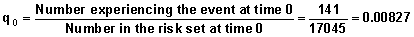Similar computations yield event probabilities for times 1, 2, . . . (See Table 6.3, column 4) Note: No calculation is performed for the duration 12+. In mortality life tables, everyone dies at the end of the life tables. However, in life tables constructed with observational data, qx) values can be calculated only up to the maximum observation period.

Step 3: Calculate survival probabilities (px)

In step 2 we calculated the probability of occurrence of an event in the indicated time. In step 3 we will calculate the complementary probability of nonoccurrence of the event at the indicated time.

Denote as px the probability that the event will not occur at the indicated time point. Then px = 1 - qx. In Table 6.3, the probability of marital disruption in the first year q0 is 0.00827. Therefore, the probability of no marital disruption in the first year (p0) = 1- 0.00827 = 0.99173. Similarly computations yield survival probabilities for all durations. (See Table 6.3, column 5.)

Step 4: Computate cumulative survival probabilities

In step 3, we calculated probabilities of nonoccurrence of the event (survival) at specific time points for those who have not experienced the event before that time point. One can also calculate a cumulative probability that an individual will not experience the event from the beginning of the observation period. For example, one can compute the probability for a newly married person not experiencing marital disruption in 7 years (or any number of years). We use the symbol S(x) to denote the probability that an individual has not experienced the event by duration x. In this example, the symbol S(7) denotes the probability that a newly married person will not experience a marital disruption in seven years.

1. Note the difference between px and S(x) . The symbol px denotes the conditional probability that a person who has not experienced the event just prior to the duration x will not experience the event at duration x. For example, the symbol p7 denotes that an individual who did not experience a marital disruption at the beginning of the seventh year of marriage will not experience a marital disruption in seventh year. In contrast to px, the symbol S(x) represents the cumulative probability an individual will not experience from the beginning of the observation period to duration x.
2. Because no one in the sample has experienced the event at the beginning of the observation period, S(0) = 1. This argument is similar to setting up l0 =1 in an ordinary life table because all people in the cohort are alive at age 0.

Because p0 denotes the probability of surviving the first duration, S(1) = p0. The probability of surviving the first two intervals will be S(2) = p0 X p1. In general the probability of surviving to the duration of x is the same as not experiencing the event at all previous durations. Therefore, S(x) can be expressed as a product as follows: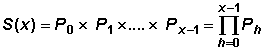The probability that an individual's marriage will be intact at duration 1 is S(1) = p0 = 0.99173. The probability that the marriage will remain intact at duration 2 is S(2) = p0 x p1 = 0.99173 x 0.98727 = 0.97911. Continue the calculations to compute the probability that the marriage will remain intact at duration 7 as follows: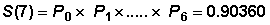The cumulative survival probabilities for all durations are displayed in Table 6.3, column 6. The survival probabilities are plotted in Figure 6.2. As expected, these probabilities decrease with time. Note: The cumulative survival probability computed as a product as done above is known in the literature as a Kaplan Meir Estimator or Product Limit Estimator.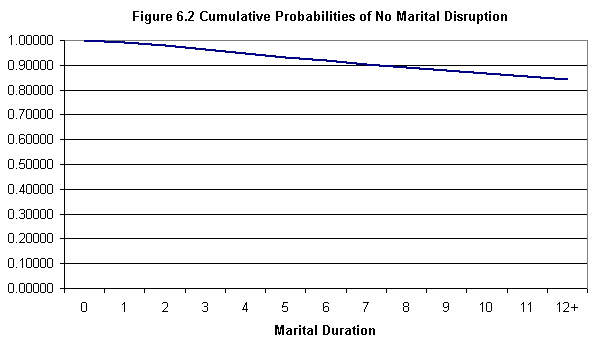Step 5: Computate the cumulative probabilities of event occurrence (cumulative probability of failure)

In step 4 we learned to compute and interpret cumulative probability of survival (nonoccurrence of the event). It is also informative to calculate the cumulative probability of the occurrence of an event by a specified duration. One may want to compute the probability that a marriage will end before seven years.

F(x) is the probability that the event will occur before duration x. In our example, F(7) denotes the probability that the marriage will end before year 7.

Once the cumulative probabilities of survival are calculated, the cumulative probability of the occurrence of the event (also called the cumulative probability of failure) can be calculated as the complement of the cumulative survival probability, i.e.,

F(x) = 1 - S(x)

In this example F(7) denotes the probability that the marriage will end before seven. It is calculated as 1 - S(7) = 1 - 0.90360 = 0.09640. From these data, we estimate that the probability that the marriage will end before seven years is 0.0964. Calculations for other durations are provided in Table 6.3, column 7. The computed cumulative probabilities of failure are plotted in Figure 6.3. The cumulative probabilities increase with the duration.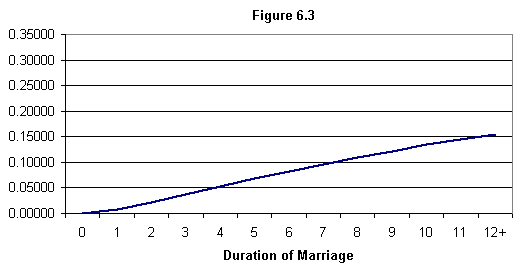A graphical representation of the cumulative probabilities of failure will illustrate the pattern of failure. The graph will be especially useful when comparing the failure times across a number of groups.

Step 6: Calculate Summary Measures

As with an ordinary life table, mean duration of time to the occurrence of the event cannot be calculated with observational data unless all of the observed individuals experience the event. Instead, the survival or cumulative probability of failure time is used to compute the median time to event. In general, one can compute the quantiles (or percentiles) of the distribution of the time to event. Note that the 25th and 75th percentiles are generally preferred.

Median duration is defined as the time at which 50% of the individuals experience the event. As shown in step 5,F(x) denotes the proportion experiencing the event by duration x. Therefore the median is the duration x when the cumulative failure rate is one half, i.e., F(x) = 0.5.

• The median is also calculated as the duration at which the cumulative survival is 0.5 or S(x) = 0.5.
• In Table 6.3, the cumulative failure rate had not reached 0.5 at duration 12, the maximum duration. Therefore, the median cannot be calculated.

The 25th and 75th percentiles are calculated in the same way as the median. For example the 25th percentile is the duration at which the cumulative failure rate is 0.25 (or the cumulative survival rate is 0.75). Similarly, the 75th percentile is the duration at which the cumulative failure rate is 0.75 (or the cumulative survival rate is 0.25).

One can calculate any percentile ranging from 0 to 100. For example, a 10th percentile is the duration at which 10% of the individuals in the life table experience the event, or alternatively, 90% do not experience the event.

 Example In Table 6.3 only 15.5% of the individuals experienced a marital disruption by year 12, the maximum period of observation. Hence, we cannot compute the median or the first or third quartiles. For illustrative purposes, we will compute the 10th percentile. In Table 6.3, the cumulative failure rate at duration 7 is 0.09640 and the rate at duration 8 is 0.10962. The 10th percentile (first decile) of the duration is the duration at which 10% experience the event, which falls between 7 and 8. The exact duration can be obtained by a simple interpolation. The first decile (10th percentile)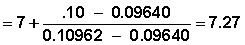Step 7: Calculate standard errors (optional)

Standard error of the conditional survival probability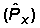The variance of the calculated survival probability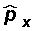is calculated as px (1 - px )/ N (x) where N(x) is the number in the risk set at duration x. The standard error is the square root of the variance.

Example

In Table 6.3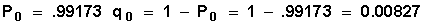N0 (Number at risk at time 0) = 17045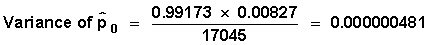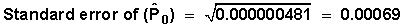Similar calculations yield variances and standard errors for all other durations. Use the Excel spreadsheet to compute the standard errors.

Duration of Marriage in Completed Years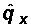Number at RiskRx VarianceStandard ErrorTable 6.4: Standard Errors of the Conditional Survival Rateor Failure Rate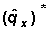of Marriage 0 0.00827 0.99173 17045 0.000000481 0.00069 1 0.01273 0.98727 16816 0.000000747 0.00086 2 0.01673 0.98327 16380 0.000001004 0.00100 3 0.01694 0.98306 15583 0.000001069 0.00103 4 0.01636 0.98364 14914 0.000001079 0.00104 5 0.01428 0.98572 14218 0.000000990 0.00099 6 0.01530 0.98470 13460 0.000001120 0.00106 7 0.01463 0.98537 12715 0.000001134 0.00106 8 0.01385 0.98615 11986 0.000001139 0.00107 9 0.01409 0.98591 11355 0.000001223 0.00111 10 0.01301 0.98699 10760 0.000001193 0.00109 11 0.001140 0.98860 10179 0.000001107 0.00105

* Standard error of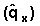is the same as standard error ofStandard Error of Cumulative Survival Probability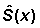Remember that the cumulative survival probabilityis estimated as the product of the conditional survival probabilities. Specifically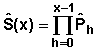Variance ofis calculated as:

Variance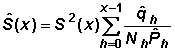For calculation purposes one can rewrite the above formula as:

Variance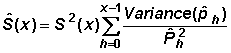The standard error ofis the square root of the variance.

Remarks

• The above formula for calculating the standard error for the cumulative survival probability is known as the Greenwood formula.
• The standard error of the cumulative failure rate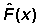is the same as the standard error of corresponding cumulative survival probability.
• Use a spreadsheet to calculate these standard errors.
 Example From Table 6.3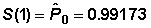Therefore, Variance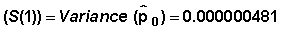The standard error of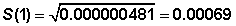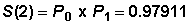Variance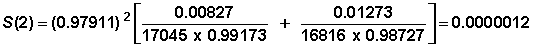The standard error of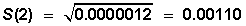Similar calculations yield standard errors for all other durations. (See Table 6.5.)

Remark

The standard error of the cumulative failure rate F(2) is equal to the standard error of S(2).

Durationof Marriagein Completed Years S(x)Number at RiskRx VarianceS(x) Standard Error Sx Table 6.5: Standard Error of the Cumulative Survival Functionof Marriage 0 1 0.99173 17045 1 0.99173 0.98727 16816 0.0000005 0.00069 2 0.97911 0.98327 16380 0.0000012 0.00110 3 0.96273 0.98306 15583 0.0000021 0.00146 4 0.94642 0.98364 14914 0.0000030 0.00175 5 0.93093 0.98572 14218 0.0000039 0.00198 6 0.91764 0.98470 13460 0.0000047 0.00216 7 .090360 0.98537 12715 0.0000055 0.00234 8 0.89038 0.98615 11986 0.0000062 0.00250 9 0.87805 0.98591 11355 0.0000070 0.00264 10 0.86568 0.98699 10760 0.0000077 0.00278 11 0.85441 0.98860 10179 0.0000084 0.00290 12 0.84468 0.0000090 0.00300

## 6.4 Construction of Life Table with Grouped Data

To construct this life table use the data from Table 6.2 on duration of pill use.

Duration of Pill Use Terminations Continuing Users Table 6.2: Duration in Months of Oral Contraceptive Use 1-34-67-910-1213-1516-1819-2122-2425-2728-3031-3334-3637-3940-4243-4546-4849+ 1095544312611814158115410513 323124242719262329132119169101336 Total 360 372

Note: In this example, the time to event is the duration of pill use. The data are grouped in intervals of unequal length (in contrast to the previous data which included exact time of event.) Slight modifications in methodology are necessary for analyzing grouped data.

Step 1: Group the data

[x, x + n] = the class interval of time to event. Here x denotes duration x and n denotes the length of class interval. In Table 6.2, data are grouped in class intervals of three (n=3) except for the last open-ended interval.

Remark

Unlike in Table 6.6, the class interval need not be the same in this type of data.

Step 2: Calculate the number at risk (not experiencing the event) at the beginning of each interval

Let Nx denote the number of individuals using the pill (individuals who have not experienced the event or who have not been censored) by duration x.

By convention, N0 will be the entire sample size (those who are exposed to the event at the beginning of the study).

Denote as ndx the number experiencing the event in the interval (x, x + n). Similarly, define nCx as the number censored in the interval.

In Table 6.3, the sample size is 732. Therefore, N0 = 732.

Among 732 women in the study, 109 stopped using the pill in the interval 1-3, and 32 were censored in the same interval. In the notation above 3d1 = 109 and 3C1 = 32. With this information we calculate the number at risk at the beginning of the next interval (4-6) as:

4 = 732 - 109 - 32 = 591

Using the notations,

N4 = N0 - 3d1 - 3C1

In general, the number at risk at the beginning of the interval is:

Nx + n = Nx - ndx - nCx

Table 6.6, column 2 displays the results of the calculated values of the number at risk at the beginning of each interval.

Duration of Pill Usein Months Nx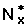nq nPn S(x) F(x) Se(nqx)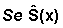Table 6.6: Life Table for Oral Contraceptive Use 1-3 732 716.0 0.15223 0.84777 1.00000 0.15223 0.01343 0.01343 4-6 591 575.5 0.09557 0.90443 0.84777 0.23325 0.01226 0.01598 7-9 505 493.0 0.08925 0.91075 0.76675 0.30169 0.01284 0.01757 10-12 437 425.0 0.07294 0.92706 0.69831 0.35262 0.01261 0.01852 13-15 382 368.5 0.07056 0.92944 0.64738 0.39830 0.01334 0.01926 16-18 329 319.5 0.03443 0.96557 0.60170 0.41901 0.01020 0.01958 19-21 299 286.0 0.02797 0.97203 0.58099 0.43527 0.00975 0.01986 22-24 265 253.5 0.05523 0.94477 0.56473 0.46645 0.01435 0.02044 25-27 228 213.5 0.07026 0.92974 0.53355 0.50394 0.01749 0.02117 28-30 184 177.5 0.04507 0.95493 0.49606 0.52630 0.01557 0.02164 31-33 163 152.5 0.07213 0.92787 0.47370 0.56047 0.02095 0.02240 34-36 131 121.5 0.04115 0.95885 0.43953 0.57855 0.01802 0.02289 37-39 107 99.0 0.04040 0.95960 0.42145 0.59558 0.01979 0.02350 40-42 87 82.5 0.12121 0.89879 0.40442 0.64460 0.03593 0.02525 43-45 68 63.0 0.07937 0.92063 0.35540 0.67281 0.03406 0.02621 46-48 53 46.5 0.02151 0.97849 0.32719 0.67985 0.02127 0.02657 49+ 39 0.32015

Step 3: Adjust the number at risk at the beginning of the interval

Because censoring (or lost to follow-up) occurs in the interval we need to make some assumptions about the pattern of censoring in each interval. Assume that censoring is uniform within each interval. Under this assumption, readjust the number at risk for the occurrence of the specific event as:

Adjusted number at risk at the beginning of the interval = Number at risk at the beginning of the interval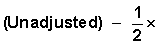Number censored in the interval.

Notation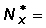Adjusted number alive at the beginning of the interval

Using the notations,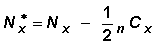Example In Table 6.6, the number at risk at the beginning of interval 1-3 is 732. Number censored in the interval is 32. Therefore the adjusted number at risk at the beginning of the interval is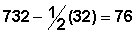We will use the notations to calculate the adjusted number at risk at the beginning of interval 7-9. Using Table 6.2 and the notations we write: N4 = 591    3d4 = 55    and    3C4 = 31 . Therefore, the adjusted number at risk at the beginning of the interval 7-9 is: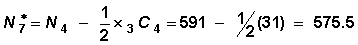Similar computations yield the adjusted number at risk for each duration in the table. (See Table 6.2, column 3).

Remarks

• Note that we do not calculate the adjusted number at risk in open-ended intervals such as 49+ in the pill use example.
• In grouped data, it is reasonable to assume that censoring is uniform throughout the interval. By this assumption, all who are censored at an event time are included in the risk set. By contrast in the marital disruption data, we assumed that event times (both complete and censored) were tabulated as the exact time of occurrence.

Step 4: Compute conditional probability of event in the interval (nqx)

Calculate the conditional probability of an event in the interval by finding the ratio of events in the interval to the adjusted number exposed. Using the notations,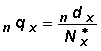Example Using data in Tables 6.1 and 6.2,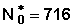and 3dt = 109. Therefore, the probability of discontinuing the use of pill in the interval 1-3 is: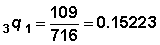Similarly, the conditional probability of discontinuing use of the pill in the interval 4-6 is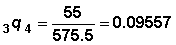Similar calculations are performed for other intervals, and the calculated probabilities are displayed in Table 6.6, column 4.

Step 5: Calculate conditional survival probabilities npx

In step 4 we calculated the probability that an individual at risk (has not experienced the event) will experience failure (or event) in the interval. The probability of not experiencing a failure (event) in the interval is the complement of the failure probability:

npx = 1 - nqx

 Example In Table 6.6 the probability of discontinuing pill use in interval (1-3) is 3q1 = 0.15223. Therefore, the probability that a woman will be a continuing user at the beginning of the interval 4-6 is: 3pt = 1 — 3q1 = 1 — 0.15223 = 0.84777 Similarly, 3P1 = 1 — 3q4 = 0.09557 = 0.90443 This number indicates that a woman who is using the pill at duration 4 will have 90.4% chance for continuing use at duration 7. Calculated values of the survival probabilities are shown in Table 6.6, column 5.

Step 6: Computate cumulative probability of survival S(x)

Calculate the cumulative probability of survival (nonoccurrence of the event) from the beginning of the observation period to the beginning of the selected interval. Denote as S(x) the probability that a person will not experience the event (failure) until duration x. Then S(x) is calculated as a Kaplan-Meier estimator as: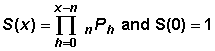Example In Table 6.6 we have already calculated the probability that a woman will continue to use the pill at month 4 is 0.84777. The probability that a woman will be a continuing user at month 7 is S(7) = 3p1 x 3p4 = .84777 x .90443 = .76675. Similar calculations yield the cumulative probabilities at other intervals. (See Table 6.2, column 6.)

Remark

When data are in grouped intervals, the cumulative survival probabilities can be calculated only to the beginning of an interval.

Step 7: Calculate cumulative probability of failure F(x)

The probability that an individual will experience the event (failure) by a specific duration is called the cumulative probability of failure. We denote this probability as F(x). This probability is the complement of the cumulative probability of survival or F(x) = 1 - S(x).

 Example In Table 6.6 the cumulative probability of survival to duration 7 (probability that a woman is continuing user of pill at month 7) is 0.76675. Therefore, the probability that a woman will stop using the pill by month 7 is 1- 0.76675 = 0.23325. In other words, there is 23% chance that a woman will stop using pill by month 7. Calculations of cumulative probabilities of failure at other durations are shown in Table 6.6, column 7.

Step 8: Compute summary measures

Compute median time to event by identifying the duration at which half of the individuals in the sample experience the event. Specifically,find the time at which the cumulative failure rate F(x) is equal to 0.5.

We can also compute the median using the estimates of the cumulative survival probabilities S(x). When F(x) is equal to 0.5, S(x) is also equal to 0.5.

One can also compute percentiles other than median. For example, the 25th percentile (or first quartile) is the duration at which F(x) = .25 or S(x) = 0.75. Similarly, the 75th percentile (third quartile) is the duration at whichF(x) = 0.75 or S(x) = 0.25.

Remark

When durations are grouped into intervals, we need to make some approximations to identify the median. Follow the example below.

 Example Calculation of median duration of pill use From Table 6.2, F(25) = 0.46645 and F(28) = 0.50394. Calculate the duration x such that F(x) = 0.5. Notice that the median falls in the interval 25-28. A simple linear interpolation can be used to approximate the median.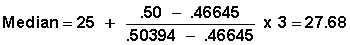In the above calculation, 3 is the length of the class interval. Calculation of the first quartile (the duration at which 25% of the study sample stop using pills) From Table 6.2, F(4) = 0.23325 and F(7) = 0.30169.Find the duration such that F(x) = 0.25. A simple linear interpolation can be performed as follows: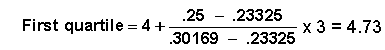Calculation of the third quartile (75th percentile) is not possible in this data set because only 68% of the woman had stopped using the pill at the maximum observation period (49 months).

Step 9: Calculate standard errors of cumulative probability of survival (or failure)

Calculate the standard errors of the conditional probability of failure (nqx) and the cumulative probability of survival (or failure). Specifically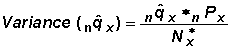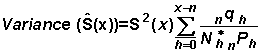(Greenwood formula)

The standard error is the square root of the variance.

 Example In Table 6.6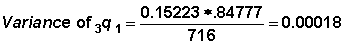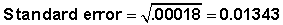Standard errors of (nqx) for each interval are given in Table 6.6, column 8. Using the Greenwood formula we can also calculate the standard error of the cumulative probability of survival (or failure) S(7) = 0.76675     (3q1 ) = 0.15223     (3p 1) = 0.84777     (3q 4) = 0.09557     (3p4) = 0.90443 Using the Greenwood formula we can compute the variance of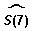as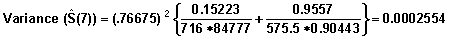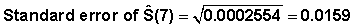Standard errors of the cumulative survival probabilities are shown in Table 6.6, column 9.

#### Exercise 1

A demographic survey interviewed 20,716 ever-married women. At the time of survey 17,269 women had at least one child in their first marriage. The remaining 3447 had experienced marital disruption of their first marriage without having a birth in the marriage or remained childless in the intact first marriage at the time of interview. (In this dataset women with premarital births are excluded.) For those who had a birth, their time to first birth in years measured from the date of marriage is noted. For those who have not experienced a birth in first marriage, the time to marital disruption (If marital disruption occurred) or to the date of survey (if in intact first marriage at the time of survey) is also recorded. The data are tabulated in Table 6.6.

Time SinceMarriage(yrs) Women with FirstBirth in the Year  Childless at Surveyor at MaritalDisruption Table 6.7: Childbirth by Duration of Marriage 0 3,871 769 1 6,263 656 2 2,854 536 3 1,560 347 4 981 270 5 602 202 6 325 155 7 238 98 8 161 81 9 102 80 10 89 62 11 65 55 12+ 158 136 Total 17,269 3,447

Assume that the marital durations to first birth are tabulated in grouped form say 0-1, 1-2, 2-3 years. Also assume that censoring is uniform in the duration interval.

Use the data to calculate:

1. Number of women (adjusted for censoring) exposed to the risk of first childbirth at the beginning of each interval
2. Probability that a woman who has not experienced a childbirth at the beginning of an interval will have a birth in the interval (qx)
3. Cumulative probability that a woman will not experience a child birth by a specified duration S(x)
4. Cumulative probability of a birth by a specified duration F(x)
5. Median time to first birth

Lesson 7: Multiple-Decrement Life Tables with Observational Data